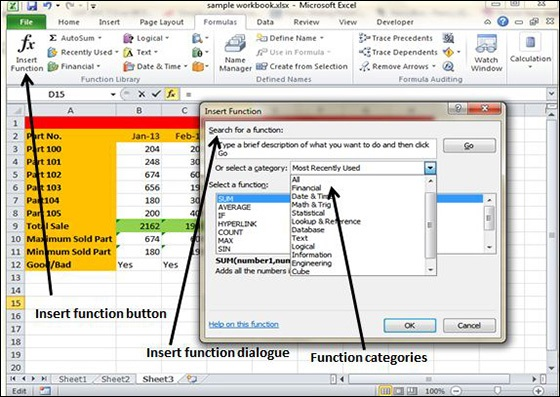# Built-in Functions in Excel 2010

## Built In Functions

MS Excel has many built in functions, which we can use in our formula. To see all the functions by category, choose Formulas Tab » Insert Function. Then Insert function Dialog appears from which we can choose the function.## Functions by Categories

Let us see some of the built in functions in MS Excel.

• Text Functions

• LOWER − Converts all characters in a supplied text string to lower case

• UPPER − Converts all characters in a supplied text string to upper case

• TRIM − Removes duplicate spaces, and spaces at the start and end of a text string

• CONCATENATE − Joins together two or more text strings.

• LEFT − Returns a specified number of characters from the start of a supplied text string.

• MID − Returns a specified number of characters from the middle of a supplied text string

• RIGHT − Returns a specified number of characters from the end of a supplied text string.

• LEN − Returns the length of a supplied text string

• FIND − Returns the position of a supplied character or text string from within a supplied text string (case-sensitive).

• Date & Time

• DATE − Returns a date, from a user-supplied year, month and day.

• TIME − Returns a time, from a user-supplied hour, minute and second.

• DATEVALUE − Converts a text string showing a date, to an integer that represents the date in Excel's date-time code.

• TIMEVALUE − Converts a text string showing a time, to a decimal that represents the time in Excel.

• NOW − Returns the current date & time.

• TODAY − Returns today's date.

• Statistical

• MAX − Returns the largest value from a list of supplied numbers.

• MIN − Returns the smallest value from a list of supplied numbers.

• AVERAGE − Returns the Average of a list of supplied numbers.

• COUNT − Returns the number of numerical values in a supplied set of cells or values.

• COUNTIF − Returns the number of cells (of a supplied range), that satisfies a given criteria.

• SUM − Returns the sum of a supplied list of numbers

• Logical

• AND − Tests a number of user-defined conditions and returns TRUE if ALL of the conditions evaluate to TRUE, or FALSE otherwise

• OR − Tests a number of user-defined conditions and returns TRUE if ANY of the conditions evaluate to TRUE, or FALSE otherwise.

• NOT − Returns a logical value that is the opposite of a user supplied logical value or expression i.e. returns FALSE if the supplied argument is TRUE and returns TRUE if the supplied argument is FAL

• Math & Trig

• ABS − Returns the absolute value (i.e. the modulus) of a supplied number.

• SIGN − Returns the sign (+1, -1 or 0) of a supplied number.

• SQRT − Returns the positive square root of a given number.

• MOD − Returns the remainder from a division between two supplied numbers.